Previous: Editing Control Points  Up: Selecting and Editing Objects  Next: Editing Text Areas

# Symmetric Shapes

A shape can have symmetry added to it using the Path Symmetry submenu of the edit path popup menu described in the previous section.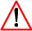Take care with closed symmetric paths. Unexpected results may occur, particularly if the path contains any gaps. This may cause the stroked or filled shape to appear unsymmetric. For example, Figure 8.8(a) shows the original (non-symmetric path). This was then given a line of symmetry and the path appears symmetric, as shown in Figure 8.8(b). In Figure 8.8(c), two of the line segments have been converted to gaps. The shape still appears symmetric although the filled area has changed, but in Figure 8.8(d) the path has been closed with a line so although the control points that make up right side of the complete path are a reflection of the original points on the left side, the shape no longer appears symmetric.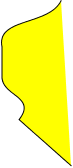(a)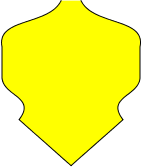(b)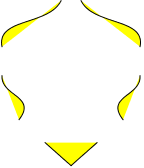(c)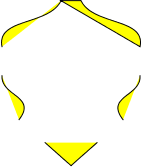(d)
Figure 8.8: Symmetric Path with Gaps: (a) the original path; (b) symmetry has been added; (c) two line segments have been replaced with gaps; (d) the path has been closed.

The Path Symmetry submenu provides the following functions:

Has Symmetry
If this menu item is selected, symmetry will be added to the path. For example, Figure 8.9(a) shows a path in edit mode, and Figure 8.9(b) shows the path with symmetry applied to it. There are now two extra control points (coloured blue). These points govern the line of symmetry8.2. In Figure 8.9(c), these two controls have been moved.

If you later decide to remove the symmetry, deselect Has Symmetry.Adding symmetry to a closed shape may cause unexpected results as the shape will be first opened (without removing the last segment), the symmetry will be added, and then the symmetric shape will be closed, merging the end points.

Anchor Join
When you add symmetry to a path, the final control point of the underlying path is anchored to the line of symmetry. That is, it can only move along the line defined by the two blue control points. To remove the constraint, deselect this menu item. In Figure 8.9(d), the Anchor Join item was deselected, and the end control was then moved away from the line of symmetry.

Note that this function places a gap (move) segment between the end control and its symmetric counterpart, which will produce an unsymmetric effect if the path is then closed. This gap can be changed to a line or curve, using Convert To Line or Convert To Curve, as described in Editing Control Points. In Figure 8.9(e), the join has been changed to a curve. Unlike the Bézier curves in the non-symmetric paths, this curve only has one curvature control.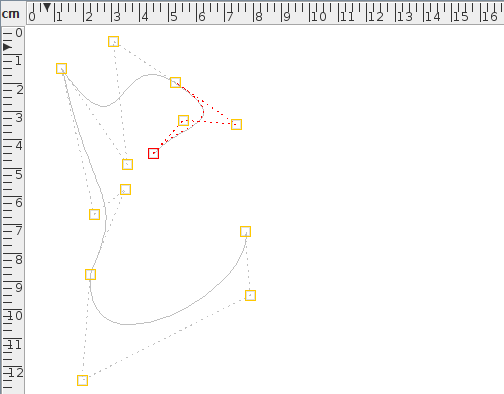(a)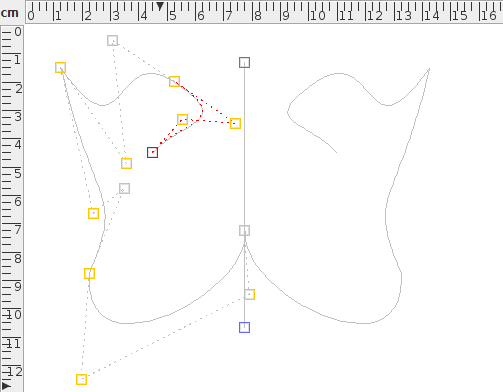(b)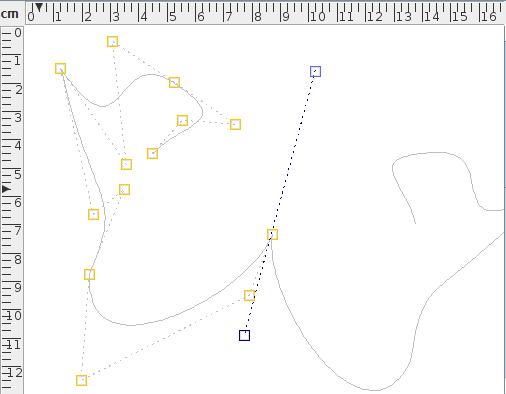(c)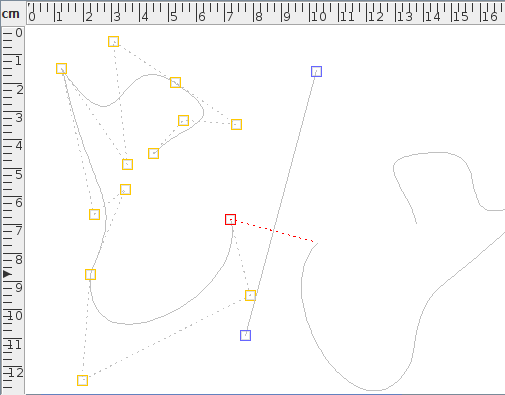(d)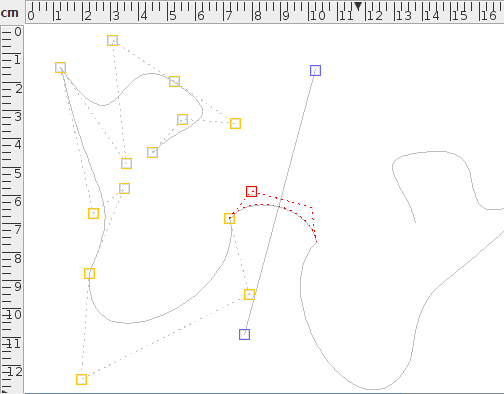(e)
Figure 8.9: Adding Symmetry to a Path: (a) original path; (b) symmetry added to path in (a) the two blue controls govern the line of symmetry; (c) the line of symmetry has been moved, altering the overall appearance of the shape; (d) the end anchor constraint has been removed and the end control has been moved away from the line of symmetry; (e) the joining segment has been converted to a curve with only one curvature control.

Anchor Start Control
This menu item is only available for closed symmetric paths. If you close a symmetric path using the Merge Ends function, the first control point of the underlying path will be anchored to the line of symmetry. The Anchor Start Control menu item will remove this constraint.

For example, Figure 8.10(a) shows a closed symmetric path (a closed version of Figure 8.9(e)). The anchor constraint on the first control was then removed and the control was moved to the left (Figure 8.10(b)). As with the join segment (above) the closing segment between the start control and its reflection can be changed to a curve with one curvature control (Figure 8.10(c)).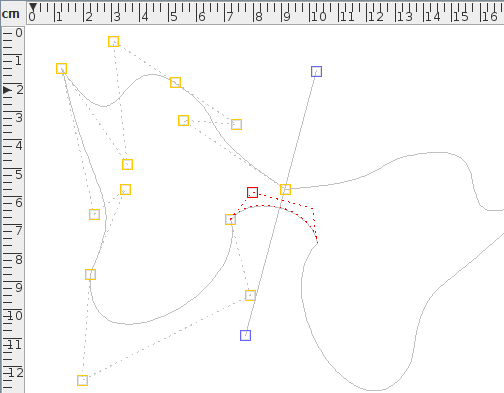(a)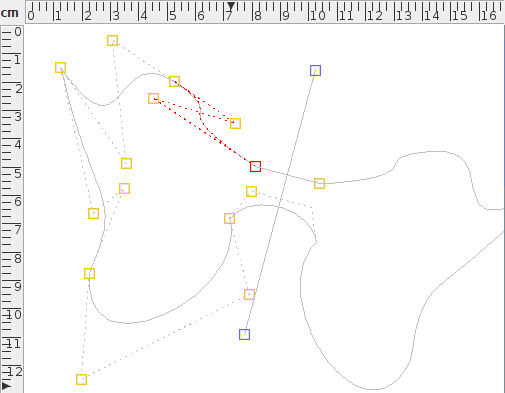(b)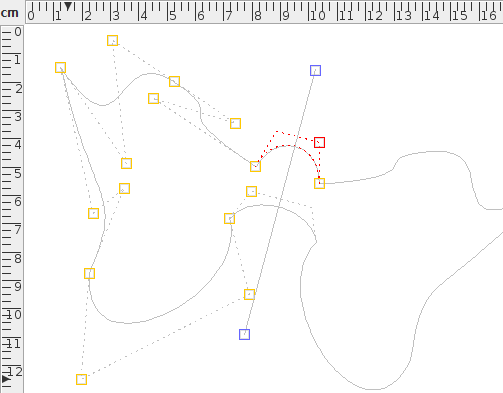(c)
Figure 8.10: Closed symmetric path: (a) the symmetric path in Figure 8.9(e) has been closed--the first control is now anchored to the line of symmetry; (b) deselecting the close anchor constraint allows the start control to be moved away from the line of symmetry; (c) the segment closing the symmetric path has been changed to a curve.